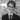# Every finite integral domain is a field.

📅December 09, 2020 | ☕️5 minutes read

Claim: Every finite integral domain is a field.

Proof: Firstly, observe that a trivial ring cannot be an integral domain, since it does not have a nonzero element. Let $F$ be our finite integral domain. By the observation above, select any nonzero element. Say $\lambda \in F$. Let $\chi : F \to F$ be a map defined by $\chi (x) = \lambda \cdot x$, where $\cdot$ is a multiplication in $F$.

Now, it suffices to show that $\chi$ is a surjective linear map. Why? If $\chi$ is surjective, then there exists $x \in F$ such that $\chi(x) = \lambda \cdot x = 1$, so $\lambda$ has a right inverse, namely $x$. But since $F$ is an integral domain, $\cdot$ is commutative, so $x$ must also be a left inverse. Hence, $\lambda$ is an unit. But since $\lambda$ was arbitrary, every element of $F$ must be an unit. Hence $F$ must be a field.

We will prove that $\chi$ is injective. Select $x, y \in F$. Suppose $\chi(x) = \chi(y)$. Now we have:

\begin{aligned} \chi(x) &= \chi(y) & \\ \lambda \cdot x &= \lambda \cdot y \\ \lambda \cdot (x - y) &= 0 \\ \end{aligned}

Now, since $\lambda \neq 0$ (by construction) and $F$ is an integral domain, we deduce $(x - y) = 0$. But then, $x = y$, so $\chi$ is indeed injective.

Now, since $F$ is finite and $\chi$ is injective, $\chi$ must be surjective. But then, by discussion in the second paragraph, $F$ must be a field. $\blacksquare$

I have decided that this proof deserves a post because it illustrates several methods in the abstract algebra. It is a good example of the power of generalisation. In this case, as it is in many others in this field, it was easier to show a more general statement. Instead of focusing how to find an inverse of an arbitrary element, we have shown $\chi$ was surjective, which is a far more general statement which helped us to push the proof forward.

It goes without saying that this, albeit a basic result, deserves a respect because it such a general statement with a remarkably elegant proof.Hi👋! I am a final year Computer Science and Mathematics student at 🎓University of Edinburgh. This is a place where I (will hopefully) write about topics I find interesting. I plan to write about my personal projects and learning experiences, as well as some random tech topics.

Built with a lot of